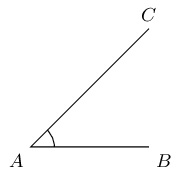Home Practice
For learners and parents For teachers and schools
Textbooks
Full catalogue
Pricing SupportLog in

We think you are located in United States. Is this correct?

# Test yourself now

High marks in science are the key to your success and future plans. Test yourself and learn more on Siyavula Practice.

# Chapter 12: Geometry of straight lines

## 12.1 Introduction

In this chapter, we will explore the relationships between pairs of angles that are created when:

• straight lines intersect (meet or cross)
• lines are perpendicular to each other
• a third line crosses two parallel linesYou will come to understand what is meant by vertically opposite angles, corresponding angles, alternate angles and co-interior angles. You will then use your knowledge to help you work out unknown angles in geometric figures.

### Notation

An angle is formed when two straight lines meet at a point. The point where the lines meet is called the vertex. Angles are labelled with a caret or 'hat' on a letter, for example, $$\hat{A}$$.

angle
the space between two straight lines that meet at a point
vertex
the point at which two straight lines meetIn this diagram, angle $$\hat{A}$$ is formed between line segments $$BA$$ and $$CA$$. We can also name the angle according to the line segments that make up the angle, for example $$C\hat{A}B$$ or $$B\hat{A}C$$.

The $$\angle$$ symbol is a short method of writing the word angle in geometry, and is often used in phrases such as "the sum of $$\angle$$s in $$\triangle$$".